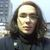Announcements
Applications are open for the 2024 Qlik Luminary Program. Apply by December 15 here.
cancel
Showing results for
Did you mean:Contributor II

## A function usage set analysis in the set analysis

Hi experts,

I'm trying to use set analysis in the set analysis, however, I'm getting an error.

My expression is like this:

=sum({<[Customer] = {"=sum({<Calendar = {">= \$(=min(Calendar))"}>} sales_amount) >= 1000000"}>} aggr(sum(sales_amount), [Customer]))

I suppose that the problem is "Calendar = {">= \$(=min(Calendar))".

Do you have any idea what correct syntax is?

1 Solution

Accepted SolutionsMVP

Try a different quote other than double quote for the inner search, like square brackets:

=sum({<[Customer] = {"=sum({<Calendar = {[>= \$(=min(Calendar))]}>} sales_amount) >= 1000000"}>} aggr(sum(sales_amount), [Customer]))

2 RepliesMVP

Try a different quote other than double quote for the inner search, like square brackets:

=sum({<[Customer] = {"=sum({<Calendar = {[>= \$(=min(Calendar))]}>} sales_amount) >= 1000000"}>} aggr(sum(sales_amount), [Customer]))Contributor II
Author

It is working, thank you so muchTags
Community Browser